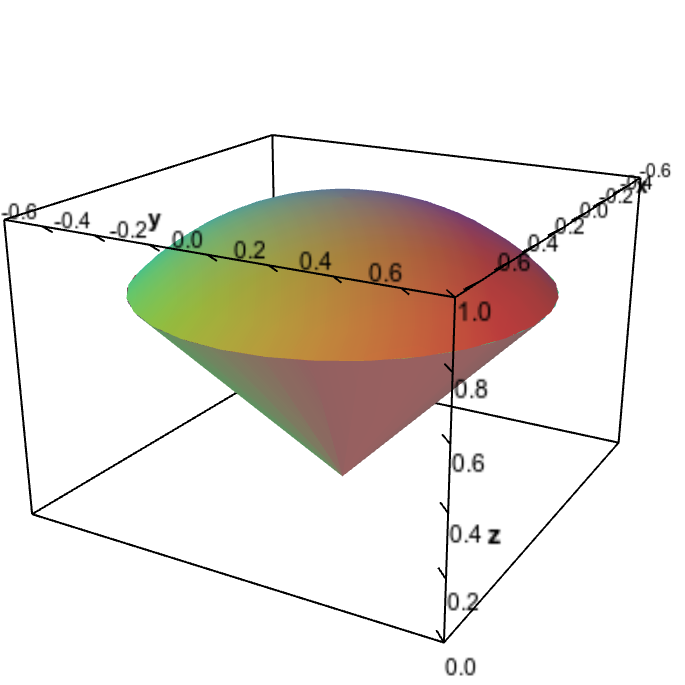# Math Insight

### Applet: Ice cream cone regionThe ice cream cone region is bounded above by the half-sphere $z=\sqrt{1-x^2-y^2}$ and bounded below by the cone $z=\sqrt{x^2+y^2}$. The two surfaces intersect along a circle defined by $x^2+y^2=1/2$ and $z=1/\sqrt{2}$.